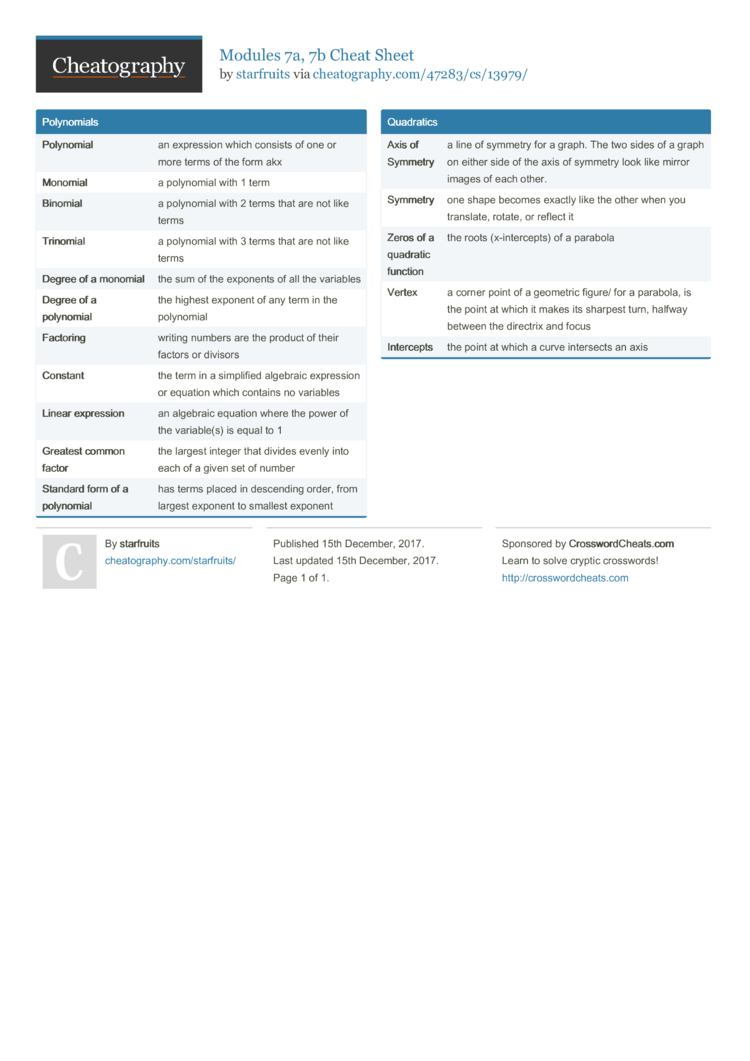# Modules 7a, 7b Cheat Sheet by starfruits

### Polyno­mials

 Polynomial an expression which consists of one or more terms of the form akx Monomial a polynomial with 1 term Binomial a polynomial with 2 terms that are not like terms Trin­omial a polynomial with 3 terms that are not like terms Degree of a monomial the sum of the exponents of all the variables Degree of a polynomial the highest exponent of any term in the polynomial Factoring writing numbers are the product of their factors or divisors Constant the term in a simplified algebraic expression or equation which contains no variables Linear expres­sion an algebraic equation where the power of the variab­le(s) is equal to 1 Greatest common factor the largest integer that divides evenly into each of a given set of number Standard form of a polyno­mial has terms placed in descending order, from largest exponent to smallest exponent

 Axis of Symmetry a line of symmetry for a graph. The two sides of a graph on either side of the axis of symmetry look like mirror images of each other. Symmetry one shape becomes exactly like the other when you translate, rotate, or reflect it Zeros of a quadratic function the roots (x-int­erc­epts) of a parabola Vertex a corner point of a geometric figure/ for a parabola, is the point at which it makes its sharpest turn, halfway between the directrix and focus Intercepts the point at which a curve intersects an axis

## Download the Modules 7a, 7b Cheat Sheet1 Page
//media.cheatography.com/storage/thumb/starfruits_modules-7a-7b.750.jpg

PDF (recommended)